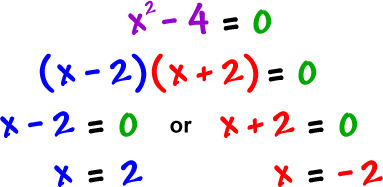Solving by Factoring
4 years ago
caityarmstrong
Save
Edit
Host a game
Live GameLive
Homework
Solo Practice
Practice12 QuestionsShow answers
• Question 1
900 secondsQ. Solve for m by factoring.
A
B
C
D
• Question 2
900 secondsQ. Solve for v by factoring.
A
B
C
D
• Question 3
900 secondsQ. Solve for x by factoring.
-6, -2
-2, 6
2, 6
-6, 2
• Question 4
900 secondsQ. Solve for v by factoring.
*Remember the equation must equal 0*
A
B
C
D
• Question 5
900 seconds
Q. Solve for a by factoring.
a2 + 2a - 24 = 0
a = -6, 4
a = 6, -4
a = 12, -2
a = 8, -3
• Question 6
900 secondsQ. Solve for n by factoring.
*Remember the equation must equal 0*
A
B
C
D
• Question 7
900 secondsQ. Solve for k by factoring.
A
B
C
D
• Question 8
900 secondsQ. Solve for x by factoring.
A
B
C
D
• Question 9
900 secondsQ. Solve for x by factoring.
A
B
C
D
• Question 10
900 secondsQ. Solve for x by factoring.
*Remember the equation must equal 0*
A
B
C
D
• Question 11
900 seconds
Q. Solve for x by factoring.
2x2 + 17x + 21 = 0
x= -21/2, -17
x= 2/3, 16
x=-7, -3/2
x=15, -3/7
• Question 12
900 secondsQ. Solve for n by factoring.